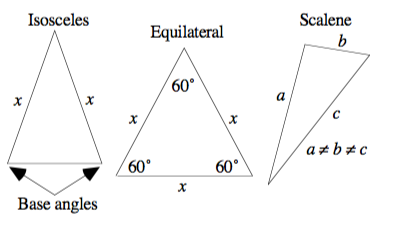# Types of triangles - Maths/数学

A triangle with two equal sides is called isosceles. The angles opposite the equal sides are called the base angles, and they are congruent (equal). A triangle with all three sides equal is called equilateral, and each angle is 60°. A triangle with no equal sides (and therefore no equal angles) is called scalene: